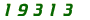Mortgage and Investment Equations

Note that for all the following equations, the interest rate is expressed as a fraction, eg 7% is 0.07. For the rate per month, divide this by 12.

Home Loan Repayments

 p is the principal a is the payment per month r is the interest rate per month n is the number of months ln is the natural log function * is multiply

Repayment amount for a set principal over a set number of payments

a = ( p * r * ( 1 + r )n ) / ( ( 1 + r)n - 1 )

Number of payments for a set principal and set repayment amount

n = ln ( a / (a - p * r ) ) / ln (1 + r)

Future Value of an Investment

 f is the future value p is the principal invested a is the amount added each month or year r is the interest rate per month or year n is the number of months or years ^ is raise to the power of * is multiply

Future value of a an amount invested for a fixed rate and time

f = p * ( 1 + r )n

Future value where a set amount is added each month or year

f = a * ( ( 1 + r )n - 1 ) / r

If you start with an initial pricipal, then add an amount each month, calculate the future value of the principal and the future value of the added amounts and add the two totals.

Where you know the initial and final amount and want the annualised return

r = ( f / p ) ^ ( 1 / n ) - 1

These financial equations are for
use in conjunction with the book

by Lorraine Graham

You can order the book in printed or e-book format through the Allen & Unwin website.

 This site is intended to supply the tools for you to do your own mortgage calculations and make your own financial decisions. The information and calculations are correct to the best of my knowledge, but are not guaranteed. Neither the author nor the publisher will accept any liability for any loss incurred from using the resources on this website.   This site was designed to look best in 800 X 600 screen resolution, but will work on any size screen.  The layout, text, backgrounds and original images used on this site are copyright © LainieJean May 02.

Site design byThis site is hosted byYou are visitor numberto the Mortgage Pages
since 1 August 2002

Web Counter provided by